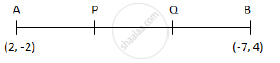# Let P and Q be the points of trisection of the line segment joining the points A(2, -2) and B(-7, 4) such that P is nearer to A. Find the coordinates of P and Q. - Mathematics

Let P and Q be the points of trisection of the line segment joining the points A(2, -2) and B(-7, 4) such that P is nearer to A. Find the coordinates of P and Q.

#### Solution

Since P and Q are the points of trisection of AB, AP = PQ = QB

Thus, P divides AB internally in the ratio 1 : 2 and Q divides AB internally in the ratio 2 : 1.∴ By section formula

P-=((1(-7)+2(2))/(1+2), (1(4)+2(-2))/(1+2))-=((-7+4)/3, (4-4)/3)-=((-3)/3,0)-=(-1,0)

Q-=((2(-7)+1(2))/(2+1),(2(4)+1(-2))/(2+1))-=((-14+2)/2, (8-2)/3)-=((-12)/3,6/3)-=(-4,2)

Concept: Section Formula
Is there an error in this question or solution?
2015-2016 (March) All India Set 1

Share